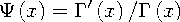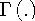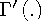Functions and CALL Routines

# DIGAMMA Function

Returns the value of the digamma function.
 Category: Mathematical

## Syntax

 DIGAMMA(argument)

### Arguments

argument

specifies a numeric constant, variable, or expression.

 Restriction: Nonpositive integers are invalid.

The DIGAMMA function returns the ratio that is given bywhereanddenote the Gamma function and its derivative, respectively. For argument>0, the DIGAMMA function is the derivative of the LGAMMA function.

SAS Statements Results
`x=digamma(1.0);`
`-0.577215665`Previous Page | Next Page | Top of Page